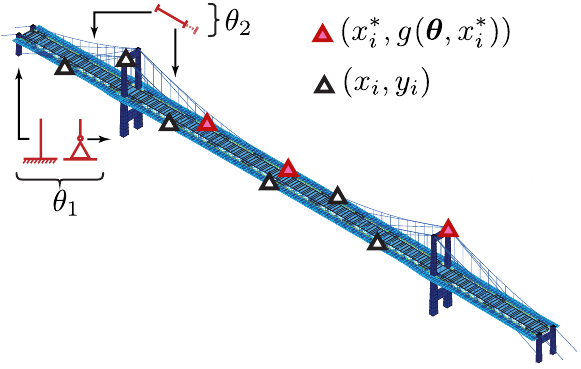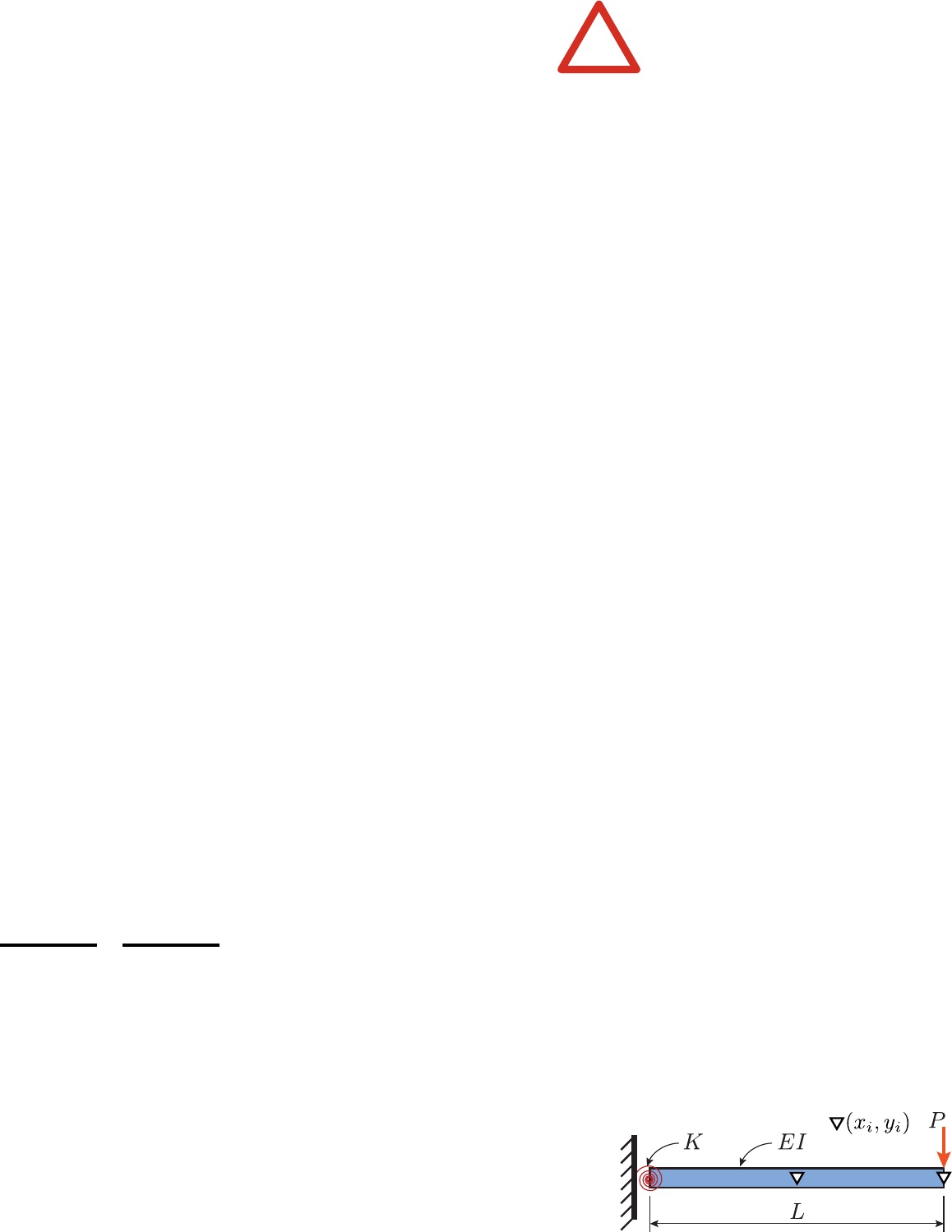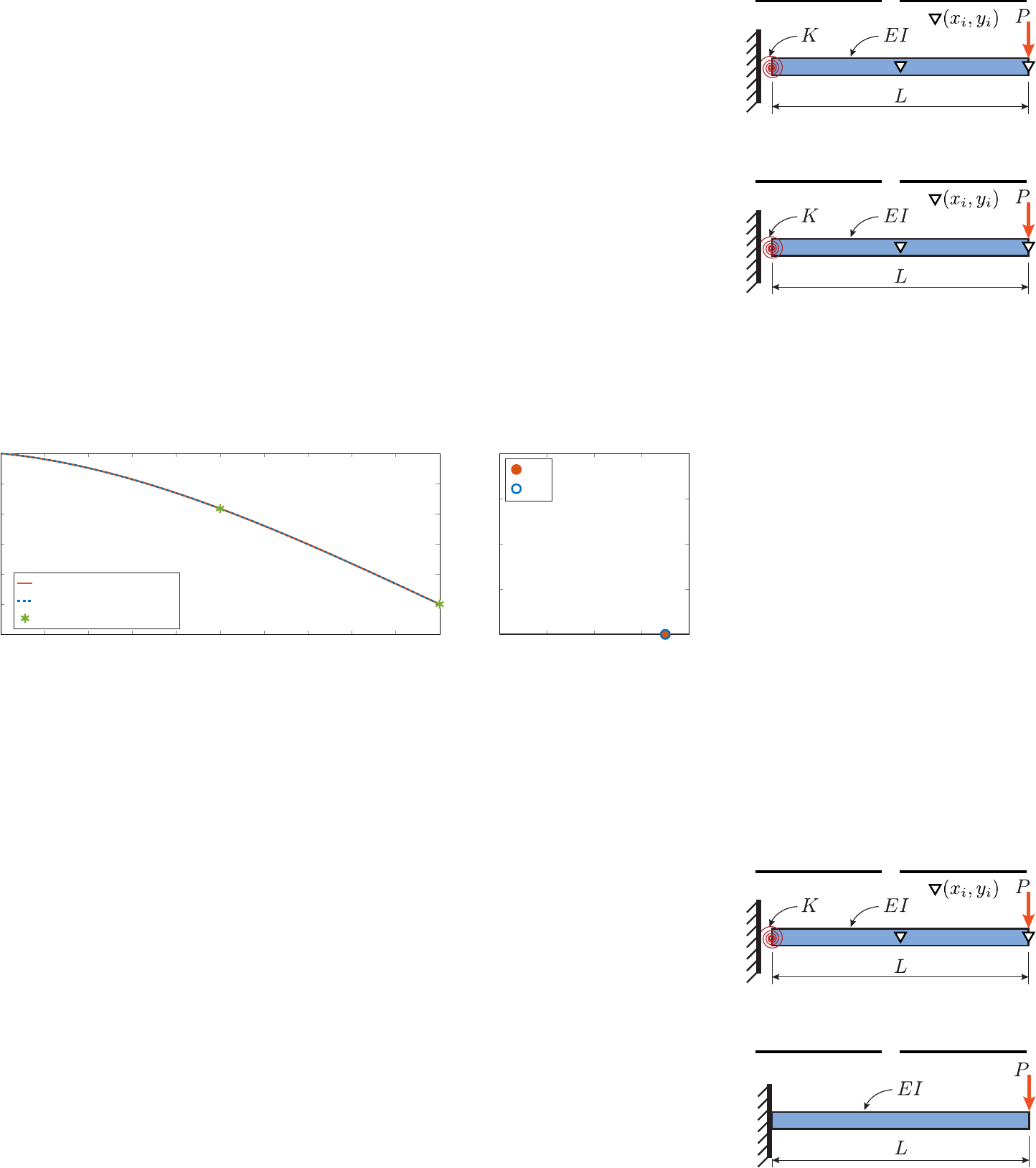13
Model Calibration
Figure 13.1: Example of hard-coded ﬁnite-
element model for which we want to infer
the parameter values
=[
1
2
]
|
from sets
of observations (x
i
,y
i
).
In civil engineering, model calibration is employed for the task of
estimating the parameters of hard-coded physics- based models using
empirical observations
D
=
{
(
x
i
,y
i
)
, 8i 2{
1:
D}}
=
{D
x
, D
y
}
.
The term hard-coded physics-based refers to mathematical models
made of constitutive laws and rules that are themselves based on
physics or domain-speciﬁc knowledge. In contrast, models presented
in chapters 8, 9, and 12 are referred to as empirical models because
they are built on empirical data with little or no hard-coded rules
associated with the underlying physics behin d the phenomena stud-
ied. The hard-coded model of a system is described by a function
g
(
, x
), which d epends on the covariat e s
x
=[
x
1
x
2
··· x
X
]
|
2 R
X
as well as its model paramete rs
=[
1
2
···
P
]
|
.Theobserved
system responses
D
y
=
{y
1
,y
2
, ··· ,y
D
}
depend on covari at es
D
x
=
{x
1
, x
2
, ··· , x
D
}
. Figure 13.1 presents an example of a model
where the parameters
describe the boundary conditions and the
initial tension in cables. For this example, the covariates
x
and
x
describe the locations where predictions are made, as well as the
type of predicti ons , t hat is, displacement, rotation, strain, and so
on. The vector
x
describes covariates for observed locations, and
x
those for the unobser ved locations.
Nomenclature
Observed system responses
D
y
= {y
1
,y
2
, ··· ,y
D
}
Covariates
D
x
= {x
1
, x
2
, ··· , x
D
}
Parameter values
=[
1
2
···
P
]
|
Model predictions
g(, x)=[g(, x
1
) ··· g(, x
D
)]
|
Model calibration is not in itself a subﬁeld of machine learning.
Nevertheless, i n th is chapter, we explore how the machine learning
methods presented in previous chapters can be employed to address
the challenges assoc i ate d wi t h the pr ob abi l i st i c in fe r en ce of mod el
parameters for hard-coded models. This application is classiﬁed
under the umbrella of unsupervised learning because, as we will
see, the main task consists in inferring hidden-state variables and
parameters that are t h ems el ves not observed.
Problem setups There are several typic al set u p s wher e we want
to employ em pi r i cal ob ser vations in conjunction wit h har d -coded
j.-a. goulet 214
models. For the example presented in ﬁgure 13.1, if we have a prior
knowledge for the joint distribution of model parameters
f
(
), we
can propagate this prior knowledge through the model in order
to quantify the unce r tai nty associated with predi c ti on s. T hi s un -
certainty propagati on can be, for instance, performed using either
Monte Carlo sampling (see
§
6.5) or ﬁrst-order linearization (see
§
3.4.2). When the uncertainty in the prior knowledge
f
(
) is weakly
informative, it may le ad t o a large variability in model predi ct i ons .
In that situation, it becomes interesting to employ empirical obser-
vations to reduce the unce r tai nty related to the prior knowledge of
model parameters. The key with model calibration is that model
parameters are typically not directly observable. For instance, in
ﬁgure 13.1 it is often not possible to directly measure the bound-
ary condition properties or to measure the cable internal tension.
These properties have t o be i n fe rr ed f r om the obse rved structural
responses
D
y
. Another key aspect is that we typically build physics-
based models
g
(
, x
) because they can predict quantities that
cannot be observed. For example, we may want to learn about
model parameters
using observations of the st at i c re sponse of a
structure deﬁned by the covariates
x
and then employ the model
to predict the unobserved responses
g
(
, x
), deﬁned by other co-
variates
x
. The vector
x
may describe unobs er ved lo cat i on s or
quantities such as the d yn ami c behavior instead of the stat i c one
employed for parameter estimation. O n e last possible problem setup
is associated with the selection of model classes for describing a
phenomenon.
There are three main challenges associated with model calibra-
tion: observation errors, prediction errors, and model complexity.
The ﬁrst challenge aris es because the observations available
D
y
are in most cases contaminated by observation errors. The second
is due to the discrepancy between the prediction of hard-coded
physics-based models
g
(
, x
) and the real system responses; that
is, even w he n par amet e r values are known, the model remains an
approximation of the re ali ty. The third challenge is related to the
diculties associated with choosing a model structure having the
right c om ple x i ty for the task at hand. In
§
13.1, we explore the
impact of these challenges on the least-squares model calibration
approach, which is stil l ex t en si vely used in practice. Then, the
subsequent sections explore how to address some of these chal-
lenges using a hierarchical Bayesian approach combining concepts
presented in chapters 6 and 8.probabilistic machine learning for civil engineers 215
13.1 Least-Squares Model Calibration
!
What we will see in
§
13.1 is an example of
what not to do when calibrating a hard-
coded physics-based model as well as why
one should avoid it.
This section presents the pitfalls and limitations of the common
least-squares deterministic model calibration. With least-squares
model calibration, the goal is to ﬁnd the model parameters
that minimize the sum of the square of the dierences between
predicted and measured values. The dierence between predicted
and observed val ue s at one obser ved location
x
i
is deﬁned as the
residual
r(, (x
i
,y
i
)) = y
i
g(, x
i
).
For the entire data set containing
D
data points, the least-squares
loss function is de ﬁn ed as
J(, D)=
D
X
i=1
r(, (x
i
,y
i
))
2
= kr(, D)k
2
.
Because of the square exponent in the loss function, its value is
positive J(, D) 0. The task of identifying the optimal parameter s
is formulated as a minimization problem where the goal is to
minimize the sum of the square of the residual at each observed
location,
= arg min
J(, D)
arg max
J(, D)
| {z }
Optimization problem
.
Gradient-based optimization methods such as those presented in
chapter 5 can be employed to i d entify
.
8
>
>
>
>
<
>
>
>
>
:
K =1.75 10
11
L =10m
P =5kN
I =6.66 10
9
mm
4
ˇ
E =35GPa
Figure 13.2: The reference model
ˇg
(
ˇ
E,x
)
that is employed to generate simulated
observations.
13.1.1 Illustrative Examples
This section presents three examples based on simulated data
using the reference beam model
ˇg
(
ˇ
E,x
) presented in ﬁgure 13.2
along with the values for its par amet e rs . O bs er vations consist in
x
1
=
L/
2 and
x
2
=
L
. The ﬁrst example corresponds to the idealized context where
there is no observation or pred i ct i on er ror s because the model
employed to infer paramet er values is the same as the reference
model employed to generat e sy nthetic data. The second example
employs a si mp li ﬁ ed model to infer parameters. Finally, the third
example employs an overcomplex model to infer parameters. Note
that the last two examples i nc l ude obse r vation errors. For all three
cases, there is a single unknown parameter: the elastic modulus
E
,
which characterizes the ﬂexural stiness of the beam.j.-a. goulet 216
Example #1: Exact model without observation errors The ﬁrst
example illustrates an idealized context where there are no obser-
vation or prediction er r ors because the model employed t o inf er
parameter values
g
(
E,x
) is the same as the one employed to gener-
ate synthetic data
ˇg
(
ˇ
E,x
). Figure 13.3 compares the predicted and
true deﬂection curves along with observed values. He re , because of
Example #1
Reference model
ˇg(
ˇ
E,x)
z }| {
Interpretation model
g(E,x)
z }| {
the absence of model or measurement er ror s, the optimal parameter
also coincides with its true value
=
E
=
ˇ
E
, and the predicted
deﬂection obtained using the optimal parameter value
coincides
with the true deﬂec t ion . Note that the los s function evaluated for
equals zero. Even if least-squares model calibrati on worked perfectly
in this example, this good performance cannot be generalized to
real case studies because in this oversimpliﬁed example, there are
no model or measureme nt errors.
0 1 2 3 4 5 6 7 8 9 10
12
10
8
6
4
2
0
Position [m]
Deﬂection [mm]
Predicted response
True deﬂection
Observations
0 10 20 30 40
0
5
10
15
20
Parameter value [GPa]
Loss function, J(, D)
E
ˇ
E
Figure 13.3: Least-squares model cali-
bration where the interpretation model
is identical to the reference model,
ˇg
(
ˇ
E,x
)=
g
(
ˇ
E,x
), and where there are
no measurement errors.
Example #2
Reference model
ˇg(
ˇ
E,x)
z }| {
Interpretation model
g(E,x)
z }| {
Example #2: S im pl iﬁ ed model with observation errors The second
example presents a more realistic case, where the model
g
(
E,x
)
employed to infer paramet er s contains a simpliﬁcation in compari-
son with the real system described by the reference model
ˇg
(
ˇ
E,x
).
In this case, the simpliﬁcation consists in omitting the rotational
spring that is responsible for the nonzero initial rotation at the
support. In addition, observations are aect e d by observation errors
v
i
: V N(v;0, 1) mm, where the observation model is d eﬁ n ed as
y
i
g(
ˇ
E,x
i
)+v
i
,V
i
?? V
j
, 8i 6= j.
Figure 13.4 compares the predicted and true deﬂection curves
along with observed values. We see that because of the combination
of model simpliﬁcation and observation errors, we obtain a higher
loss for the correct parameter value
ˇ
E
= 35 GPa than for the
optimal value
E
= 22 GPa. As a result, the model predictions
are also biased for any prediction location
x
. This second example
illustrates how deterministic least-squares model calibration may
identify wrong parameter values as well as lead to inaccurate
predictions for measured and unmeasured locations.